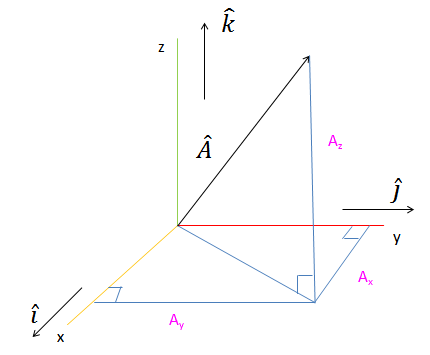# Three Dimensional Vector Calculation

Calculate the magnitude of three dimensional vectors (3D Vectors) for entered vector coordinates.

 Vector A: x y z

The 3D vectors are using the x-y-z axes. The vector OP has initial point at the origin O (0, 0, 0) and terminal point at P (2, 4, 5). So we can draw the vector OP . We saw above that the distance between 2 points in 3-dimensional space is distance AB=√(x2−x1)2+(y2−y1)2+(z2−z1)2Let consider the standard (x,y,z) cartesian coordinate system in three dimensional space, starting with a origin point and lets construct three mutually perpendicular axes, which are x-axis, y-axis, and z-axis. These axes are depicted in the figure labeled as x, y, and z. (in the case of negative value of axis will be draw in the opposite side of the origin, where the axes intersect.)

So we can drawn the vector A or say OP .

We saw above that the distance between 2 points in 3-dimensional space is distance AB=√(x2−x1)2+(y2−y1)2+(z2−z1)2

Since we assumed originating point O as (0,0,0) and terminal point at P (2, 4, 5) for the vector A, therefore the magnitude of the vector is given by:

∣OP∣=√22+42+52

= √4+16+25

=√45

= 6.708 units